Open In App

# How to calculate a square root?

The square root of any numerical value is a value that on self multiplication results in the original number. ’√’ is the radical symbol used to depict the root of any number. By square root, we mean a power 1/2 of that number. For instance, let us suppose that x is the square root of any integer y, this implies that x=√y. On multiplying the eq, we also obtain x2 = y.

The square root of the square of a positive number gives the original number.

To understand the concept, we know, the square of 4 is 16, and the square root of 16, √16 = 4. Now, as we can see, 16 is a perfect square figure. This makes it easy to compute the square root of such numbers. However, to compute the square root of an imperfect square like 3, 5, 7, etc, computing root is a difficult process.

A square root function is a one-to-one function that uses as input a positive number and returns the square root of the given input number.

f(x) = √x

Properties of Square Roots:

Some of the important properties of the square root are as follows:

• For a perfect square number, a perfect square root exists.
• For a number ending with an even number of zeros, a square root exists.
• The square root of any negative numbers is not defined.
• For a number ending with the digits 2, 3, 7, or 8, then the perfect square root does not exist.
• For a number ending with the digits 1, 4, 5, 6, or 9, then the number will have a square root.

### How to calculate a square root?

Perfect square numbers are integers that are positive in nature and can be easily expressed in the form of the multiplication of a number by itself. Perfect square numbers are depicted as the value of power 2 of any integer. Computation of square root of perfect square numbers is relatively easier. There are primarily four methods used to find the square root of numbers:

• Repeated Subtraction Method of Square Root
• Square Root by Prime Factorization Method
• Square Root by Estimation Method
• Square Root by Long Division Method

The above three methods can be used in the computation of the square root of perfect square numbers. The last method, however, can be used for both types of numbers.

### Repeated Subtraction Method of Square Roots

The method relies on the following sequence of steps:

Step 1: Subtract consecutive odd numbers from the number for which we are finding the square root.

Step 2: Repeat step 1 until a value of 0 is attained.

Step 3: The number of times step 1 is repeated is the required square root of the given number.

Note: This method can be used only for perfect squares.

For instance, for the number 16, the method works as follows:

16 – 1 = 15

15 – 3 =12

12 – 5 = 7

7- 7 = 0

The process is repeated 4 times. Thus,√16 = 4.

### Square Root by Prime Factorization Method

Prime factorization of any number is the representation of that number in the form of a product of prime numbers. The method relies on the following sequence of steps :

Step 1: Divide the specified number into its prime factors.

Step 2: A pair of similar factors is formed in a way such that both the factors in each of the formed pairs are equal.

Step 3: Take one factor from each of the pairs.

Step 4: The product of the factors is obtained by taking one factor from each pair.

Step 5: This obtained product is the square root of the given number.

Note: This method can be used only for perfect squares.

For instance, for the number 64, the method works as follows:64 = {2 × 2} × {2 × 2} × {2 × 2}

64 = 22 × 22 × 22

64 = (2 × 2 × 2)2

64 = (8)2

√64 = 8

### Square Root by Estimation Method

The estimation method is used for approximating the square root of a given number. It approximates the square root of a number to a reasonable guess of the actual value. Calculations are easier in this method. However, it is a really long and time taking process.

Step 1: Find the nearest perfect square occurring both before and after to the given number.

Step 2: Find the next closest integers and round them off each time to come towards the closest answer.

For instance, for the number 15, the method works as follows:

9 and 16 are the perfect square numbers before and after nearest to 15. Now, we know,

√16 = 4 and √9 = 3. This implies that the square root of the number 15 occurs between 3 and 4. Now, the process involves the evaluation of whether the square root of the number 15 is closer to 3 or 4.

The first case is taking 3.5 and 4. Square of 3.5 = 12.25 and the square root of 4 = 16. Therefore, the square root of integer 15 lies between 3.5 and 4 and is closer to 4.

Further, we find the squares of 3.8 and 3.9, which are equivalent to 3.82 = 14.44 and 3.92 = 15.21 respectively. This implies that √15 lies between 3.8 and 3.9. On further evaluation, we obtain that √15 = 3.872.

### Square Root by Long Division Method

The Long Division method for the computation of the square root of numbers involves the division of large numbers into steps or parts, thus breaking the problem into a sequence of easier steps.

For instance, for the number 180, the method works as follows:

Step 1: A bar is placed over every pair of digits of the number beginning with the unit’s place.

Step 2: The left-most number is then divided by the largest number such that the square is less than or equal to the number in the left-most pair.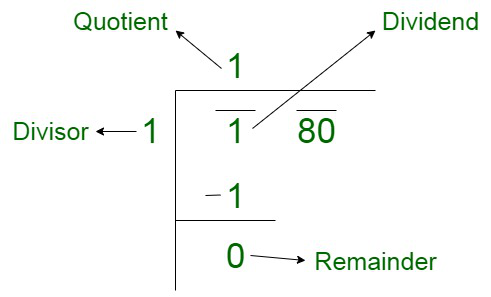Step 3: Now, the number under the next bar to the right of the remainder is brought down. The last digit of the quotient obtained is added to the divisor. Now, the next step is to find a number to the right of the obtained sum, such that it together with the result of the sum, forms a new divisor for the new dividend.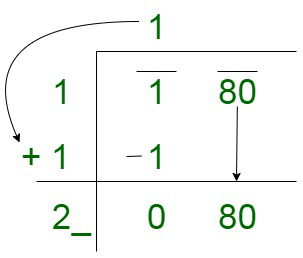Step 4: The obtained number in the quotient is equivalent to the number as selected in the divisor.

Step 5: The same process is repeated using a decimal point and adding zeros in pairs to the remainder.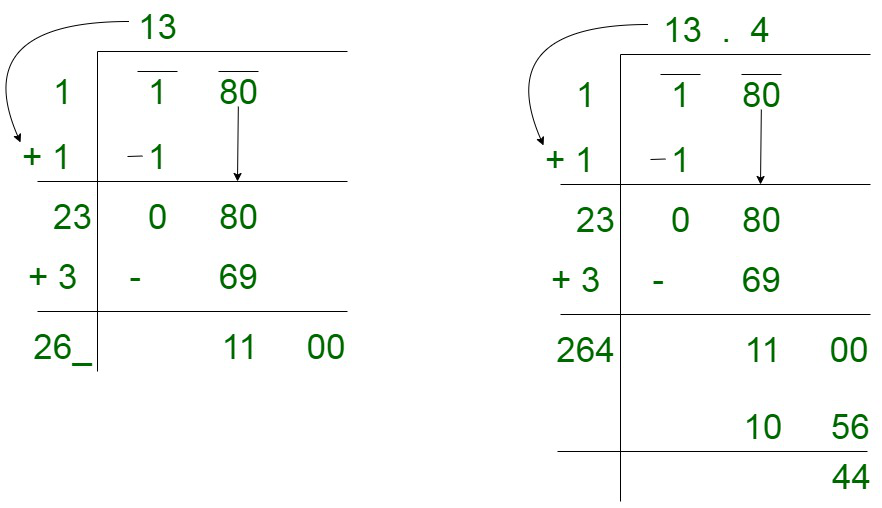Step 6: The quotient forms the square root of the number.

### Sample Questions

Question 1. Compute the square root of 144 by Prime Factorization Method?

Solution:144 = {2 × 2} × {2 × 2} × {3 × 3}

144 = 22 × 22 × 32

144 = (2 × 2 × 3)2

144 = (12)2

√144 = 12

Question 2. What is the way to simplify square root?

Solution:

The prime factorization of the given number can be computed. In case the factor cannot be grouped, a square root symbol is used to group them. The following rule is used for simplification :

√xy = √(x × y), where, x and y are positive integers.

For instance, √12 == 2√3

In case of fractions, the following rule is used:For example:= √5

Question 3. Solve: √(x + 2) = 4

Solution:

We know,

√(x + 2) = 4

On squaring both the sides, we obtain;

x + 2 = √4

x + 2 = ±4

x = ±4 – 2

Therefore, we have,

x = 2 or x = -6

Question 4. Can the square root of a negative number be a whole number? Explain.

Solution:

We know, the negative numbers cannot have a square root. The reason behind this is that if two negative numbers are multiplied together, the result obtained will always be a positive number. Therefore, the square root of a negative number will be in the form of complex number.

Question 5. Compute the square root of 25 by the method of repeated subtraction?

Solution:

Going by the above stated steps, we have,

25 – 1 = 24

24 – 3 = 21

21 – 5 = 16

16 – 7 = 9

9 – 9 = 0

Since the process is repeated 5 times, therefore, we have,√25 = 5.

Question 6. Compute the square root of 484 by the long division method?

Solution:

By the long division method, we have,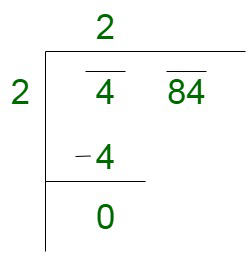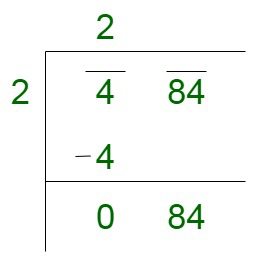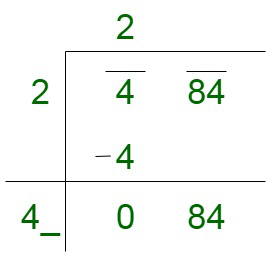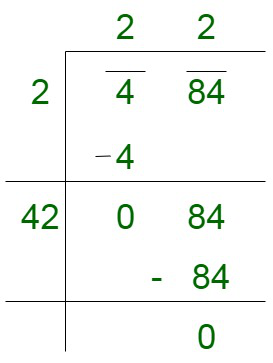Now,

The remainder is 0, therefore, 484 is a perfect square number, such that,

√484 = 22